# 2nd PUC Physics Previous Year Question Paper March 2017

Students can Download 2nd PUC Physics Previous Year Question Paper March 2017, Karnataka 2nd PUC Physics Model Question Papers with Answers helps you to revise the complete Karnataka State Board Syllabus and score more marks in your examinations.

## Karnataka 2nd PUC Physics Previous Year Question Paper March 2017

Time: 3 Hrs 15 Min
Max. Marks: 70

Instructions:

1. All parts are compulsory.
2. Answer without relevant diagram/figure/circuit wherever necessary will not carry any marks.
3. Numerical problems solved without writing the relevant formulae carry no marks.

Part – A

I. Answer all questions. Each question carries one mark. (10 × 1 = 10)

Question 1.
State Coulomb’s law.
Coulomb’s law: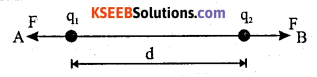‘The Electrostatic force of attraction or repulsion between two stationary point charges separated in free space or air is directly proportional to the product of magnitudes of charge on them and inversely proportional to the square of the distance between them and the force acts along the line joining the two point charges’.
F = $$\left(\frac{1}{4 \pi \varepsilon_{0}}\right)\left(\frac{q_{1} q_{2}}{d^{2}}\right)$$N.

Question 2.
Define mobility of electron.
Electron mobility is defined as the ratio of drift velocity of electron to the applied electric field.Question 3.
What is the significance of Lenz’s law?
Lenz’s law is based on the law of conservation of energy.

Question 4.
Define dispalcement current.
Current in a capacitor is called displacement current.

Question 5.
Write one application of microwave.
Microwaves are used in aircraft navigation, detection of speeds of fast balls, tennis serves and automobiles. Microwaves are used in micro oven in which water molecules resonate resulting in the raise of temperature of food containing water.

Question 6.
How is the power of a lens related to its focal length?
The power of a lens is measured as the reciprocal of the focal length of a lens.

Question 7.
Write an expression for de-Broglie wavelength of a particle.
The de-Broglie’s equation is λ = $$\frac{h}{m v}$$, where λ is the de Broglie wavelength, m the mass and v the velocity of the particle.Question 8.
What is the conclusion of Davisson – Germer experiment of the nature of electron?
Davisson – Germer experiment proves the wave nature of electron in motion.

Question 9.
Write the SI unit of activity.
Becquerel is the SI unit of radioactivity.

Question 10.
What is transducer in communication system?
A transducer converts one form of energy into another.

Part – B

II. Answer any five of the following questions: (5 × 2 = 10)

Question 11.
Write any two properties of electric field lines.

1. Electric lines of force never intersect with each other.
2. Electric lines of force exert lateral stress on one another.

Question 12.
On what factors does the capacitance of a parallel plate capacitor depends?
Capacitance of a parallel plate capacitor depends directly on the area of cross section of the plate, dielectric medium between the two plates and inversely proportional to the thickness of the dielectric medium.Question 13.
State and explain Ohm’s law.
Ohm’s law: When all the physical conditions surrounding the conductor remain unaltered, the electric current in a conductor is directly proportional to the potential difference between its ends.

Question 14.
Define the terms:

1. Declination
2. Inclination or Dip.

1. The angle between the magnetic meridian and the geographic meridian is known as the magnetic declination.
2. The angle between the earth’s magnetic meridian (horizontal component of earth’s field) and the earth’s total magnetic field is known as the angle of dip.

Question 15.
State and explain Faraday’s law of electromagnetic induction.
It states that whenever there is a change in the magnetic flux linked with a circuit an emf is induced in it and the magnitude of the induced emf is directly proportional to the rate of change of magnetic flux.

Question 16.
Name the type of lens used to correct.

1. Myopia
2. Hypermetropia.

1. Concave Lens
2. Convex Lens

Question 17.
What is NAND gate? Give its logic symbol.
A NAND gate is a negated AND gate in which the output will remain low if and only if both the inputs are high.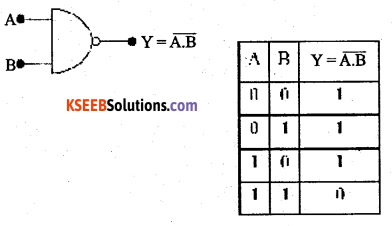Question 18.
Draw the block diagram of a AM receiver.Part – C

III. Answer any five of the following questions. (5 × 3 = 15)

Question 19.
Derive the relation between electric field and electric potential due a point charge.
Consider a point charge ‘+q’ Let ‘P’ be a point initially at infinity. Let ‘A’ be a point inside the . field region. Let ‘+1C’ be a unit positive charge moved from the point at infinity to point ‘A’. Let ‘A’ be at a distance of ‘r’ from the given point charge. Let ‘B’ be another point at distance of’dr’, from’A’towards the point charge +q.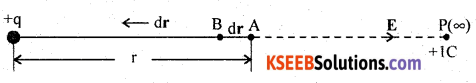The work done in moving positive test charge from A to B, against the force of repulsion is dW = – F.dr. The -ve sign indicates that the work is done against the direction of the force and dr is the displacement of+1C of test charge in the direction opposite to the electric field.
But electric potential dV = $$\frac{d W}{+q_{0}}$$ and electric field E = $$\frac{\mathrm{F}}{+q_{0}}$$
When q0 = +1C then dW = dV, E = F
Hence dV = -Edr or E = $$-\frac{d \mathrm{V}}{d r}$$
i.e., Electric field intensity at a point is the negative potential gradient at that point and electric field intensity is in the direction of decreasing electrostatic potential.

Question 20.
Derive an expression for energy stored in a charged capacitor.
Let ‘C’ be the capacitance of a parallel plate capacitor. By definition C = $$\frac{Q}{V}$$. By definition amount of work done to raise the charge by ‘dq’ is dW = V dq.
i.e., dW= $$\frac{q}{C}$$dq
where ‘q’ is the instantaneous value of charge on the conductor.
Total work done in charging the capacitor is given by,or W = $$\frac{1}{2} \frac{Q^{2}}{C}$$, amount of work done is stored in the form of energy.
Therefore E = $$\frac{1}{2}\left(\frac{Q^{2}}{C}\right)$$
Using Q = CV, E = $$\frac{1}{2}$$ CV2, where ‘V’ is the maximum voltage supplied to a capacitor.Question 21.
Explain with circuit diagram how to convert galavanometer into a voltmeter.To convert a galvanometer into a voltmeter, a high resistance is connected in series with the coil. The galvanometer with this modification is called a voltmeter. The value of the high resistance R to be connected in series with the coil depends on the maximum potential difference to be measured. If Ig be the current for a full scale deflection then,
V = Ig(G + R)
G is the resistance of the galvanometer.
∴ R = $$\frac{V}{I_{g}}$$ – G
Ig ∝ V
Since G and R are constants, the scale can be graduated to read potential differences directly. A voltmeter is used for measurement of potential difference. It should be connected in parallel in a circuit.

Question 22.
Derive an expression for motional emf induced in a condctor moving in a uniform magnetic field.Let ‘PQ’ represent a conductor moving with a speed V at right angles to the magnetic flux density ‘B’ pointing perpendicular to the sheet of paper and pointing towards the paper. Let RQ = x be the distance covered by the conductor. Magnetic flux ΦB = Blx.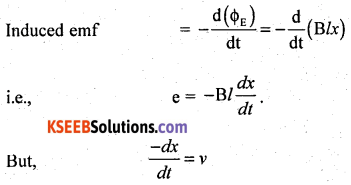∴ e = Blv ________(1)
Note: The Lorentz force acts on a charge in a conductor and acts along PQ. Work done in moving a charge ‘q’ from P to Q is
W = (Lorentz force) (displacement of the charge ‘q’)
i.e., W = (Bqv)l
by definition electric p.d = $$\frac{\mathrm{W}}{\boldsymbol{q}}=\frac{\mathrm{B} q v l}{q}$$ = Bvl _________(2)
Equation (1) and (2) are identical.Question 23.
What is a transformer? Mention two sources of energy loss in a transformer.
A transformer is a device used for stepping up or stepping down the AC voltages. Sources of energy loss in transformers are due to

1. Copper loss
3. Hysteresis loss
4. Eddy current loss.

Question 24.
Mention any three applications of polaroids.
1. Polaroids are used to produce and detect plane polarised light.
2. Polaroids are used in photographic cameras to eliminate unwanted portion of light.
3. Polaroids are used as microscope objectives to eliminate glare of light from the minute particles under observation.

Other applications:
4. Polaroids are used in the construction of Laurentz half shade polarimeter.
5. Polaroids are used to improve the colour contrast in the old paintings.

Question 25.
Write any three experimental observations of photoelectric effect.
Photo electric effect is used in the,

1. Reproduction of sound in motion pictures.
2. Automatic opening and closing of doors.
3. Thickness control of paper in the paper manufacturing.
4. Television transmission.Question 26.
Give any three differences between p-type and n-type semiconductors.

 p-type Semiconductors n-type Semiconductors 1. Pure semiconductor is doped with elements of III group (acceptors) Pure semiconductor is doped with elements of V group (donors) 2. Holes are majority charge carriers Electrons are majority charge carriers. 3. Energy levels of dopants is above the upper limit of valence band. Energy levels of dopants is below the lower limit of conduction band.

Part – D

IV. Answer any two of the following questions: (2 × 5 = 10)

Question 27.
Deduce the condition for balance of a Wheatstone’s bridge using Kirchhoff s rules.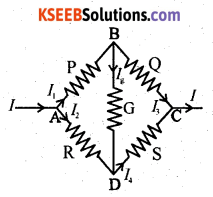Applying the KCL to junction B and D,
I1 = I3 + Ig ________(1)
I4 = I2 + Ig ________(2)
Applying the KVL to the mesh ABDA,
I1P + IgG – I2R = 0
i.e; I1P + IgG = I2 R ______(3)
Applying the KVL to the mesh BCDB,
I3Q – I4S – IgG = 0 ______(4)
For a balanced condition of Wheatstone’s network, Ig = 0.
∴ (1) and (2) may be written as I1 = I3 and I2 = I4
(3) and (4) may be written as
I1P = I2R _______(5)
and I3Q = I4S _____(6)
(5) ÷ (6) gives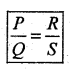Question 28.
Derive an expression for magnetic field at a point on the axis of a circular current loop.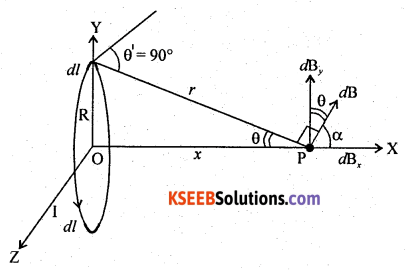Let R be the radius of a current loop, carrying current I. Let R be a point on the axis of a conductor. Let dB be magnetic field at P, due to a current element ‘idl’.
From the figure, θ + α = 90°, so that α = 90° – θ
and cos α = cos (90° – θ) = sin θ = $$\frac{R^{2}}{\left(R^{2}+x^{2}\right)^{\frac{1}{2}}}$$ _______(1)
Let dBx be the horizontal component of dB.
Applying Biot-Savart’s law,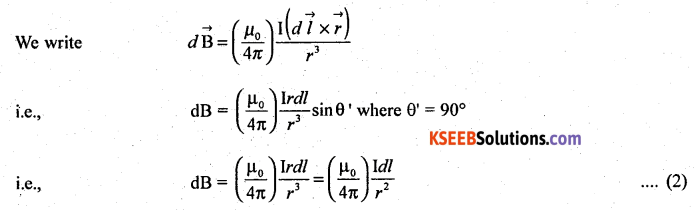Component of dB along the horizontal is dBx = dBcos α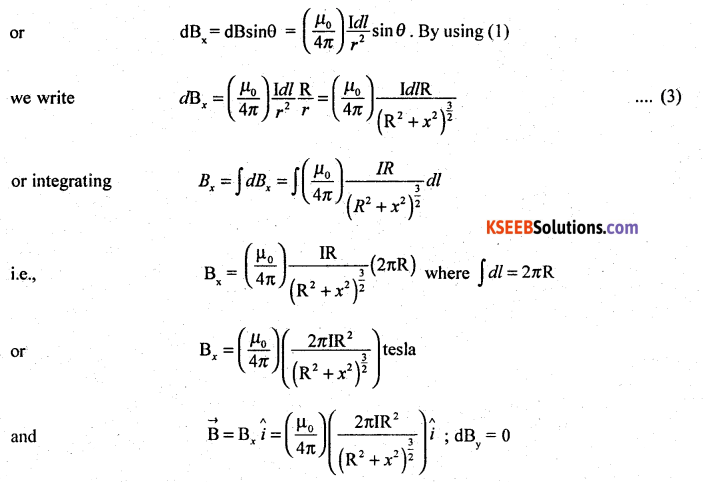For a circular loop, and at the centre, x = 0.For a circular conductor containing n turns,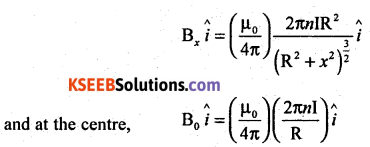Question 29.
Write any five properties of ferromagnetic materials.

1. These are slightly attracted towards a strong magnetic field.
2. These substances exhibit the properties like magnetostriction and hysteresis.
3. These substances obey Curie Weiss law χ ∝ $$\frac{1}{\mathrm{T}-\mathrm{T}_{c}}$$.
4. Magnetic permeability is far greater than one.
5. Magnetic susceptibility is very large (10 to 106).

V. Answer any Two of the following questions: (2 × 5 = 10)Question 30.
Derive Lens Maker’s, formula for a convex lens.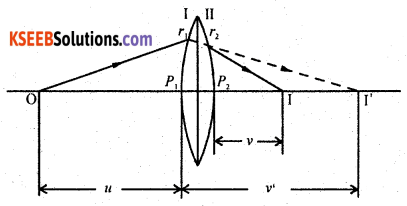Let r1 and r2 be the radii of curvature of the thin lens. Let O be the point object at a distance u from the pole of the first curved surface of the lens. Real image is formed at I’, at a distance v’ from the pole P1. This image is formed in the denser medium.
We know that from the refraction formula,where n1 is the refractive index of rarer medium and n2 that of the denser medium (n2 > n1). For the second surface, real image at I will serve as a virtual object and the distance is taken as -ve. The object space is the lens medium for refraction through the second curved surface. Final image is formed in air at I and at a distance of V from P2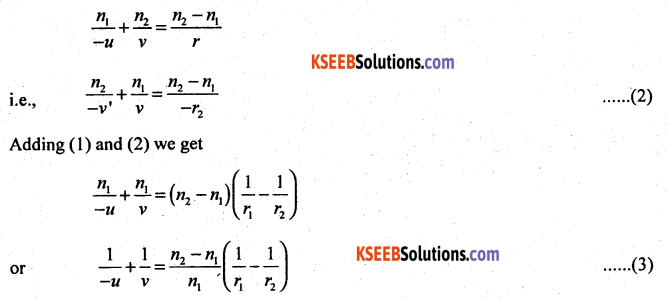where u = ∞, v = f
when u = f, v = ∞
∴ The term on the L.H.S. can be replaced by $$\frac{1}{f}$$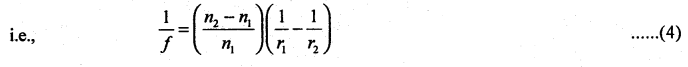where f is the focal length of lens.
The equation (4) is called the lens maker’s formula.Note:
(1) Using the equation (4), it can be shown that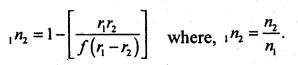(2) For radius of curvature the letter ‘R’ may be used.

Question 31.
State radioactive decay law. Derive N = N0e-λt for a radioactive element.
The rate of disintegration of ratio active atoms present in the sample of an element is directly proportional to the number of radioactive atoms present at that instant.
i.e; $$\frac{d N}{d t}$$ ∝ N
or $$\frac{d v}{d t}$$ = – λN
where ‘λ’ is known as the disintegration constant. The -ve sign indicates that the number of radio active nuelei/atoms decreases with the passage of time.
Hence, $$\frac{d N}{N}$$ = – λ dt
Integration both sides we get
loge = – λ t + CApplying the initial condition for t = 0, N = N0
We get, C = loge N0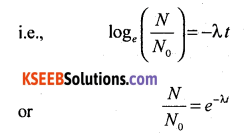Hence, N = N0e-λt
A graph ‘N’ v/s time ‘t’ gives the exponential curve as shown in the figure.Question 32.
What is rectification? With relevant circuit diagram and waveforms explain the working of p-n junction diode as a full wave rectifier.
Full wave rectification:
The process in which both the halves of a.c. are rectified to obtain a pulsating direct current is known as full wave rectification.

A transformer with a centre tap is used for a full wave rectification. Input contains ac. A minimum of two diodes are used at the secondary of the transformer. A load resistor is connected between the junction of the N type semiconductor and the centre tap.

During the first half cycle, diode D1 will be forward biased and D2 will be reverse biased so , that the current flows from B to centre tap.During the second half cycle, diode, D2 will be forward biased while D1 will be reverse biased. The current once again takes the same direction from B to centre tap. The I/P and the O/P waveforms are shown in fig. The average DC is 2$$\left(\frac{V_{p}}{\pi}\right)$$.VI. Answer any three of the following questions: (3 × 5 = 15)Question 33.
Two point charges qA = 3μC and qB = 3μC located 20 cm apart in vacuum.

1. What is the electric field at the mid point O of the line AB joining the two charges?
2. If a negative test charge of a magnitude 1.5 × 10-9 C is placed at this point, then , What is the force experienced by the test charge?

Given qA = 3 × 10-6 C, qB =- 3 × 10-6C, r = 0.20 m
1. Electric field at the midpoint ‘0’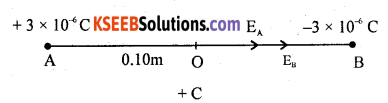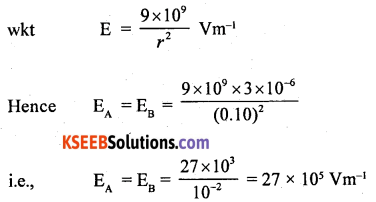The direction along AB or OB.
∴ Net electric field at = EA + EB
= 5.4 × 106 Vm-1 along OB

2. Net force on -1.5 × 10-9 C placed at ‘0’
F = q0E0
i.e., F = -1.5 × 10-9 × 5.4 × 106 N
= 8.10 × 10-3 N in the direction along OA.Question 34.
When two resistors are connected in series with a cell of emf 2V and negligible internal resistance, a current of $$\frac{2}{5}$$ A flows in the circuit. When the resistors are connected in parallel the main current is $$\frac{5}{3}$$ A. Calculate the resistances.
Given E = 2V, r = 0, IS = $$\frac{2}{5}$$ A
IP = $$\frac{5}{3}$$ A, R1 = ?, R2 = ?
1. Resistors in series: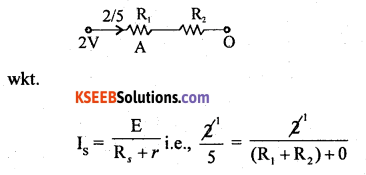i.e; R1 + R2 = 5 ________(1)

2. Resistors in parallel:∴ R1 + R2 = 5; R1R2 = 6
and R1 + R2 = $$\sqrt{(5)^{2}-4(6)}$$ = 1 _______(2)
Solving R1 + R2 = 5
R1 – R2 = 1
2R1 = 6
R1 = 3Ω and 3 – R1 = 1
∴ R2 = 2Ω
Hence R1 = 3Ω, R2 = 2Ω.Question 35.
A source of alternating emf of 220 V – 50 Hz is connected in series with a resitance of 200Ω an inductance of 100 mH and a capacitance of 30μF. Does the current lead or lag the voltage and by what angle?
Given, Vrms = 200V, f = 50 Hz, R = 200Ω
L = 100 × 10-3 = 0.1 H, C = 30 × 10-6 F = 3 × 10-5 F Interactive rectance XL = 2πfL
i.e., XL = 2 × 3.142 × 50 × 0.1Ω
i.e., XL =31.42Ω
Capacitive rectance XC = $$\frac{1}{2 \pi f C}$$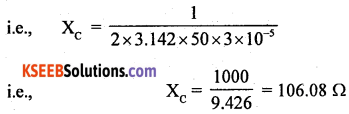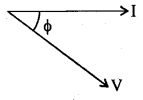Reactence of the circuit XL – XC = 31.42 – 106.08
i.e; XL – XC = -74.6Ω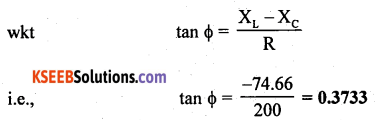Hence f = -20°30′
i.e., the current leads the voltage by 20° 30′.Question 36.
Light of wave length 6000 A° is used to obtain interference fringes of width 6 mm in a Young’s double slit experiment. Calculate the wave length of light required to obtian fringe of width 4 mm when the distance between the screen and slits is reduced to half of its initial value.
Given λ = 6800 × 10-10, m = 6 × 10-7 m
λ1 =?
β = 6 × 10-3 m, β1 = 4 × 10-3 m
D1 = D/2
w.k.t fringe width β = $$\frac{\lambda \mathrm{D}}{d}$$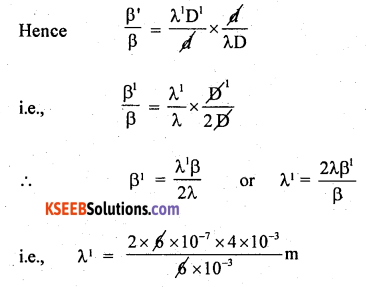λ1 = 8 × 10-7
or λ1 = 8000 Å
The wavelength required to produce fringes of width 4 mm is 8000 A°.Question 37.
The first member of the Balmer series of hydrogen atom has wavelength of 6563A°. Calculate the wavelength and frequency of the second member of the same series. Given c = 3 × 108 ms-1.
Given λα = 6563 A°= 6.536 × 10-7 m
λβ = ?, fβ = ?
c = 3 × 108 ms-1
wkt, $$\frac{1}{\lambda}$$ = R$$\left(\frac{1}{n_{1}^{2}}-\frac{1}{n_{2}^{2}}\right)$$.
For I member of balmer series, n1 = 2, n2 = 3i.e; R = 1.097 × 107 m-1
For II member of Balmer series
x1 = 2, x2 = 4i.e; λ2 = 4.8617 × 10-7m
or λ2 = 4.8617 A°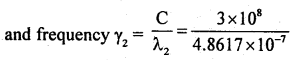i.e; γ2 = 0.6171 × 1015 Hz = 6.171 × 1014 Hz.

error: Content is protected !!